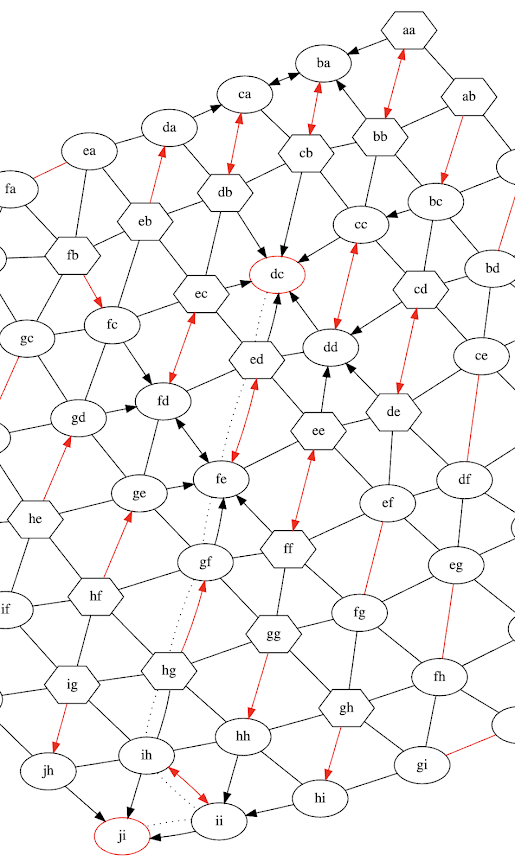### Another Treatment of Odd Cycles in the Blossom Algorithm

Blossom algorithm is an algorithm for constructing maximum matchings on any indirected graphs. This algorithm tries to find an augmenting path between two unmatched vertices. An augmenting path having alternative matched and unmatched edges, while both end vertices must be unmatched. If we could find an augmenting path, it is easy to replace all matched edges with the unmatched edges to increase the matched size (edge by 1 and vertices by 2).

The algorithm maintains a forest of vertices, while all unmatched vertices are the single root at the start and unmatched edges and matched edges are added in pair to one tree. In the forest, each vertex is marked as even (root and deeper vertices of matched edges) or odd.  If we find an unmatched edge to join two even vertices belonging to two different trees, we find an augmenting path.

In the forest construction, we ignore edges which would add a loop. But this may cause missing of some critical alternative paths. We do not need to track all alternative paths up to the tree's root, but it is important to track both even and odd paths if both existing.

So we only need treat odd cycles, as even loop keeps vertices even and odd.

a -- b == c -- x == y
\-- u == v

In the above simplified case,  '--' is for unmatched edges and '==' is for matched edges. The vertices a, c, y and v are even. An edge between y and v will make y and v also odd.

Edmonds found that we could contract the odd cycle into a single vertex and restart the augmenting process. An augmenting path in the contracted graph could be lifted to the original graph easily. It is also easy to show that this contraction keeps the existence of augmenting path.

Most pseudo code description of this algorithm uses a hard restart after the contraction. This is not effective. The implementation in Boost.Graph is better. It uses a disjoint set to represent the contraction and keep the existing forest.

In this article, we describe another treatment. In the forest, each matched vertices could be even or odd. If we use two links (may be null) for even and odd parents separately. The links for the above odd cycle could be

x ==> y --> v ==> u --> c ==> b --> a
u ==> v --> y ==> x --> c ==> b --> a

The x is an even vertex in the first path, while it is an odd vertex in the second path.

The same as before, we must avoid loops in the same parity path. It is a little tricky that the nearest ancestor may be odd now if there are multiple odd cycles share some edges. We must stop the reversing links at the nearest ancestor to avoid loops.

Demo

In this demo, we use red for matched edges. Edges in the forest are directed up to unmatched roots. Vertices having a red shape are unmatched roots. Vertices having a hexagon shape are pending (even and to be visited).

vertex=ad

expand ad -- ac == bd

link odd cycle ad -- bd == ac

vertex=ea

expand ea -- da == eb
odd cycle ea <-> eb

link odd cycle ea -- eb == da
odd cycle relinked ea <-> eb

vertex=cd

expand cd -- cc == dd

find augment path
vertex=ea

expand ea -- da == eb
odd cycle ea <-> eb

link odd cycle ea -- eb == da
odd cycle relinked ea <-> eb

vertex=ba

expand ba -- aa == bb
expand ba -- cb == dc
expand ba -- ca == db
odd cycle ba <-> bb

link odd cycle ba -- bb == aa
odd cycle relinked ba <-> bb

vertex=eb

already odd linked eb .. da

Another demo for a larger grid